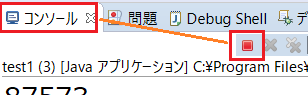# Java While Statement Sample

This is a sample of repeating a process with Java's while statement.

## What is the while statement?while (conditions){       Process to be executed }
• Repeats the internal process while the condition is true.
• If the condition is false, the loop process will be terminated.
• If the first conditional decision is false, the loop will not execute even once.
• Note that if there is no logic in the process to set the condition to false, it will result in an infinite loop.

### Sample while statement

``````public class Test1 {
public static void main(String[] args) {
int i = 0;

while (i < 5) {
System.out.println(i); // 0,1,2,3,4
i++;
}
}
}``````

Line 5 repeats the process while the value is less than 5.
The seventh line adds the value that is the condition.

### Exiting the loop(break)

``````  int i = 0;

while(true){
if (i == 3) {
break;
}
System.out.println(i); //0,1,2
i++;
}``````

The break in line 5 exits the while statement.
The third line sets the condition to true. Note that this sample will become an infinite loop without the break in line 5.

### Exit the double loop(label)

``````  int i = 0;
while1: while (true) {
while (true) {
if (i == 3) {
break while1;
}
System.out.println(i); // 0,1,2
i++;
}
}
System.out.println("end"); // end``````

It is a double loop with two while's, where while1 is a label.
The break and label in line 5 breaks the outer while statement.

``````  int i = 0;

while(i < 5){
if (i == 3) {
i++;
continue;
}
System.out.println(i); //0,1,2,4
i++;
}``````

The continuation of line 6 executes line 3 next.
Note that in this sample, if there is no addition in line 5, it will become an infinite loop.

### How to stop an infinite loop (when using Eclipse)

If you encounter an infinite loop when using Eclipse, press the red square icon in the "Console" tab. (Eclipse 4.8)Related Articles

△上に戻る